# Abstract

A combination of vectorial form of wave method (VWM) with Fourier expansion series is proposed as a new vehicle for free and forced vibration analysis of stepped cylindrical shells with multiple intermediate flexible supports. The flexible supports can include springs with arbitrary properties in the possible directions. Based on Flügge thin shell theory and VWM, the reflection, propagation, and transmission matrices for a circular cylindrical shell are defined. Furthermore, contiguous vector-matrix relationships are established for free and forced vibration analysis of the issue including an arbitrary number of the discontinuities in the shell thickness, or shell steps, and intermediate supports. Using these vector-matrix relations, the equations of motion as well as the system continuity are well satisfied. Dimension of these vectors and matrices are completely, independent of the number of the applied supports and geometrical steps in the shell. Hence, the present approach provides excellent computational advantages and modeling flexibility compared to the conventional vibration analysis methods available in the literature. The results of the present study are compared with the results available in the literature as well as the results of finite element method (FEM) and found in excellence agreement. Furthermore, as a case study case, a cylindrical shell with three flexible intermediate supports and also three geometrical steps is considered. The natural frequency and mode shapes of the issue are derived, and the forced responses of the shell subject to point load excitation are reported.

Keywords:
Intermediate flexible supports; stepped cylindrical shell; extended vectorial-wave method; free and forced vibration

# 1 INTRODUCTION

Using intermediate supports for long pipe uniform or non-uniform shells in many industries is a common way for protecting the system from the occurrence of resonance failure and improving the system robustness. In this regard, rigidity consideration for the supports applied, called classical supports, is an over simplified assumption bring significant deviations between the theoretical and experimental results. It is clear that as much as the characteristics of non-rigid supports can play a great rule on variation of the responses of the whole vibrating system. In addition, existence of discontinuity in the shell thickness or shell steps is inevitable in many industrial elements such as accumulators, fluid tanks and pipes. Hence, following the importance of the support characteristics and the non-uniform thickness of the shell in the practical application, the researchers have been encouraged to examine these effects on the vibration analysis of shells like in Zhou et al. (2012Zhou, H., Li, W., Lv, B., Li, W.L. (2012). Free vibration of cylindrical shells with elastic-support boundary conditions, Applied Acoustics 73: 751-756.), Qu et al. (2013Qu, Y., Chen, Y., Long, X., Hua, H., Meng, G. (2013). Free and forced vibration analysis of uniform and stepped circular cylindrical shells using a domain decomposition method, Applied Acoustics 74: 425-439.), Chen et al. (2015Chen, M., Xie, K., Xu, K., Yu, P., Yan, Y. (2015). Wave based method for free and forced vibration analysis of cylindrical shells with discontinuity in thickness, ASME Journal of Vibration and Acoustics 107: 051004, 1-14.), Chen et al. (2013Chen, Y., Jin, G., Liu, Z. (2013). Free vibration analysis of circular cylindrical shell with nonuniform elastic boundary conditions, International Journal of Mechanical Sciences 74: 120-132.), and finally Wang et al. (1997Wang, C. M., Swaddiwuhipong, S., Tian, J. (1997). Ritz method for vibration analysis of cylindrical shells with ring stiffeners, Journal of Engineering Mechanics, 123(2):134-142.). In this way, different numerical methods, e.g. finite element method (FEM) like in Salahifar and Mohareb (2012Salahifar, R., Mohareb, M. (2012). Finite element for cylindrical thin shells under harmonic forces, Finite Elements in Analysis and Design, 52: 83-92.), weighted residual method as in Qu et al. (2013Qu, Y., Chen, Y., Long, X., Hua, H., Meng, G. (2013). Free and forced vibration analysis of uniform and stepped circular cylindrical shells using a domain decomposition method, Applied Acoustics 74: 425-439.), generalized differential quadrature method (GDQ) as in Loy et al. (1997T. Loy, C., Y. Lam, K., Shu, C. (1997). Analysis of cylindrical shells using generalized differential quadrature, Shock and Vibration 4: 193-198.), and analytical solutions, e.g. close form solutions as those represented by Chen et al. (2013Chen, Y., Jin, G., Liu, Z. (2013). Free vibration analysis of circular cylindrical shell with nonuniform elastic boundary conditions, International Journal of Mechanical Sciences 74: 120-132.), wave based method (WBM) like in Chen et al. (2015Chen, M., Xie, K., Xu, K., Yu, P., Yan, Y. (2015). Wave based method for free and forced vibration analysis of cylindrical shells with discontinuity in thickness, ASME Journal of Vibration and Acoustics 107: 051004, 1-14.), transfer function method (TFM) as in Zhou et al. (1995Zhou, J., Yang, B. (1995). Distributed transfer function method for analysis of cylindrical shells, AIAA Journal 33: 1698-1708.) and state space techniques (SST) like in Zhang and Xiang (2007Zhang, L., Xiang, Y. (2007). Exact solution for vibration of stepped circular cylindrical shells, Journal of sound and vibration 299: 948-964.), are proposed for analysis of such systems.

Analytical modeling of vibrations of uniform shells with interior supports has been a critical issue in the recent analytical studies, perhaps due to requirement of satisfying the continuity and the support conditions simultaneously, like in Qatu (2002Qatu, M.S. (1989-2000). Recent research advances In the dynamic behavior of shells, (2002) Part 2: Homogeneous Shells. ASME Applied Mechanics Reviews, 55: 415-434.), Xiang et al. (2002Xiang, Y., Ma, Y.F., Kitipornchai, S. (2002). Exact solutions for vibration of cylindrical shells with intermediate ring supports, International Journal of Mechanical Sciences 44: 1907-1924.), Zhang and Xiang (2006Zhang, L., Xiang, Y. (2006). Vibration of open circular cylindrical shells with intermediate ring supports, International Journal of Solids and Structures 43: 3705-3722.) and Loy and Lam (1997Loy, C.T., Lam, K.Y. (1997). Vibration of cylindrical shells with ring support, International Journal of Impact Engineering, 35 (1997) 455-471.). Hence, a few researches been conducted in this area, and their proposed methods are numerated and contain several limitations. For example Xiang et al. (2002Xiang, Y., Ma, Y.F., Kitipornchai, S. (2002). Exact solutions for vibration of cylindrical shells with intermediate ring supports, International Journal of Mechanical Sciences 44: 1907-1924.) solved the issue of free vibrations of a uniform shell with several intermediate ring-, or radial rigid-supports. They proposed a state-space technique combined with domain decomposition method in order to satisfy continuity as well as support conditions of the issue. In this direction, Zhang and Xiang (2006Zhang, L., Xiang, Y. (2006). Vibration of open circular cylindrical shells with intermediate ring supports, International Journal of Solids and Structures 43: 3705-3722.) with the same methodology proposed in Xiang et al. (2002Xiang, Y., Ma, Y.F., Kitipornchai, S. (2002). Exact solutions for vibration of cylindrical shells with intermediate ring supports, International Journal of Mechanical Sciences 44: 1907-1924.), solved the problem of free vibrations of a uniform open shell with multiple intermediate Ring Supports (RS). Although the results of the method proposed in Xiang et al. (2002Xiang, Y., Ma, Y.F., Kitipornchai, S. (2002). Exact solutions for vibration of cylindrical shells with intermediate ring supports, International Journal of Mechanical Sciences 44: 1907-1924.) are promising as an analytical method, the applied intermediate RS were not flexible and were only limited in the one direction, i.e. radial direction. The vibration analysis of stepped shells and modeling of the flexible supports are possible using the numerical methods, e.g. FEM where proposed in Qu et al. (2013Qu, Y., Chen, Y., Long, X., Hua, H., Meng, G. (2013). Free and forced vibration analysis of uniform and stepped circular cylindrical shells using a domain decomposition method, Applied Acoustics 74: 425-439.). However, solving the problem demands several degrees of freedom for the issue and accordingly extensive memory resources and high computational resources (this important evaluated in Qu et al. (2013Qu, Y., Chen, Y., Long, X., Hua, H., Meng, G. (2013). Free and forced vibration analysis of uniform and stepped circular cylindrical shells using a domain decomposition method, Applied Acoustics 74: 425-439.)). Moreover, in special applications such as designing with optimization, systems controls, inverse problems, and real time applications, accurate and fast system responses as well as low system memory are required simultaneously. Thus, in the mentioned applications, applying the conventional and efficient numerical methods like FEM may fail to capture the shell response within a reasonable computational time and accuracy.

In contrast with the numerical methods that demand high memory and computational costs, the analytical approaches requires not only low memories but also low computational costs. However, as mentioned, the available analytical methods are deal with major limitation on introducing flexible intermediate supports. Hence, it is clear that developing new approaches which are capable of providing robust, fast, and accurate results for vibration analysis of shells, is highly demanded to deal with the design problems with less limitations and more capabilities.

Vectorial wave method (VWM) is a well-known method, which was first introduced by Mace (1984Mace, B.R. (1984). Wave reflection and transmission in beams, Journal of sound and vibration, 97(2):237-246.) and applied to free and forced vibration analysis in straight beams. Thereafter, VWM was applied in vibration analysis of several wave guide elements such as bars, Hagedorn and Das-Gupta (2007Hagedorn, P., Das-Gupta, A. (2007). Vibrations and waves in continuous mechanical systems, John Wiley & Sons Ltd, England.), straight-, Mase (2005), and curved-beams and frames in two,Lee et al. (2007Lee, S.-K., Mase, B.R., Brennan, M.J. (2007). Wave propagation, reflection and transmission in curved Beams, Journal of Sound and Vibration 309:639-656.), or three dimensions, Mei (2016Mei, C. (2016). Experimental validation of wave vibration analysis of complex vibrations in a two-story metallic space frame based on the Timoshenko bending theory. ASME Journal of Vibration and Acoustics 138(2):021003.), rings, Huang et al. (2013Huang, D., Tang, L., Cao, R. (2013). Free vibration analysis of planar rotating rings by wave propagation. Journal of Sound and Vibration 332:4979-4997.), plates, Ma et al. (2015Ma, Y., Zhang, Y., Kennedy, D. (2015). A symplectic analytical wave based method for the wave propagation and steady state forced vibration of rectangular thin plates, Journal of Sound and Vibration 339:196-214.) and Renno and Mace (2011Renno, J.M., Mace, B.R. (2011). Calculating the forced response of two-dimensional homogeneous media using the wave and finite element method, Journal of sound and vibration, 330: 5913-5927.), and membranes, Bahrami et al. (2012Bahrami, A., Ilkhani, M.R., Nikkhah-Bahram, M. (2012). Wave propagation technique for free vibration analysis of annular circular and sectorial membranes. Journal of Vibration and Control 0:1-7.). However, this method has not been extended for other mechanical elements such as shell like geometries.

Review of the literature shows that there have been few attempts for extension of VWM to more complex issues such as existence of multiple geometrical steps or multiple intermediate supports in considered geometry. Nikkhah-Bahrami et al. (2011Nikkhah-Bahrami, M., Khoshbayani-Arani, M., Rasekh-Saleh, N. (2011). Modified wave approach for calculation of natural frequencies and mode shapes in arbitrary non-uniform beams, Scientia Iranica 18(B):1088-1094.) have proposed a modified form of VWM for free vibration analysis of non-uniform mechanical elements (beams) with arbitrary numbers of discontinuities. They demonstrated that by distinctive combinations of VWM matrices and their inversions, it is possible to provide a reduced order vector-matrix relationship, independent of number of discontinuity of the considered issue. However, due to singularity of the reflection and transmission matrices in the vicinity of rigid intermediate supports, the proposed method in Nikkhah-Bahrami et al. (2011Nikkhah-Bahrami, M., Khoshbayani-Arani, M., Rasekh-Saleh, N. (2011). Modified wave approach for calculation of natural frequencies and mode shapes in arbitrary non-uniform beams, Scientia Iranica 18(B):1088-1094.), cannot be extended to the issues with intermediate supports. To the authors' best knowledge which is supported by a critical literature survey, the VWM has not yet been utilized for vibration analysis of shell elements or problems with interior supports perhabs due to lack of establishment of proper wave vectors and relevant matrices.

The present study aims to extend VWM method to the case of stepped cylindrical shell elements with interior flexible supports, as barriers in the direction of the wave dispersion. Hence, proper wave vectors and relevant matrices relationships for these kinds of wave barrier are stablished. By introducing a canonical relationship between carrier waves and the displacement field, a general case study including possible barriers in the path of wave motion, flexible intermediate support types and geometrical steps, are considered. Base on carrier wave vector motion in each shell segment and communication between the waves in all the shell interfaces, extended VWM is utilized for free and forced vibration analysis of cylindrical shells with discontinuities in their thickness in the presence of several intermediate flexible supports.

# 2 GOVERNING EQUATIONS

Figure 1 shows a cylindrical shell with the length L, thickness h, Poisson ratio μ, Young's modulus E, density ϱ and middle layer radius a. The governing equations of motion based on Flügge theory for a cylindrical shell as demonstrated in Figure 1 can be expressed as follows:

(1)where, Lij (i,j=1,2,3) are differential operators as shown in appendix A. Furthermore, ux, ϑ, r are the spatial displacements of the shell middle layer in the axial, x, circumferential, ϑ, and radial, r, directions, respectively. Using time harmonic functions and complex Fourier expansion in the circumferential direction, as to satisfy Eq. (1), the displacement fields can be represented in the respective directions as:

(2)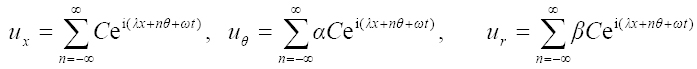where, C is a constant; α and β are displacement ratios, which are respectively introduced as α=uθ /ux and β=ur /ux . In Eq. (2), λ is the wave number, n is the circumferential mode number (or integer constant of the Fourier terms) and ω is circular frequency. In fact, Eq. (2) consists of the displacement field regarding to all possible real and imaginary excitation responses of the shell. To obtain real parts of the calculated results, a half-range expansions of the Fourier series is enough for the issue, i.e. n=0 to ∞. To establish the parameters of λ and ω, Eq. (2) is substituted in the equation of motion, Eq. (1), which yields:

(3)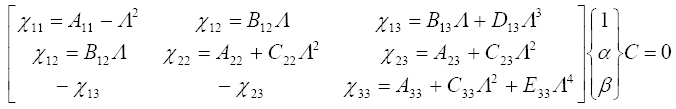where Λ is a dimensionless wave number as Λ=λa, and χij (i,j=1,2,3) are the matrix elements. The expressions (A,B,C,D)ij (i,j=1,2,3) are given in Appendix B. To achieve the non-trivial results and hence the non-zero displacement fields, the determinant of the coefficients matrix in Eq. (3) should be equal to zero, i.e. | χij |=0.

By defining a dimensionless frequency Ω=ωa((1-μ 2)ϱ/E)0.5 (or frequency factor) as the matrix square eigenvalues [ χij ], we can achieve a logical relationship among the dimensionless wave numbers Λ and frequency factor Ω with the aid of | χij |=0. These relations are established for all kind of velocities of wave's dispersion in the shell, i.e. the phase velocity and the group velocity of the waves (Lee et al. (2007Lee, S.-K., Mase, B.R., Brennan, M.J. (2007). Wave propagation, reflection and transmission in curved Beams, Journal of Sound and Vibration 309:639-656.) and Karczub (2006Karczub, D.G. (2006). Expression for direct evaluation of wave number in cylindrical shell vibration studies using the Flügge equation of motion, Journal of the Acoustical Society of America 119:3553-3557.)) where this issue will not be discussed here. Mathematically, |χij |=0 leads to a polynomial of degree four in terms of square of dimensionless wave number Λ 2 as follow:

(4)in which Ai (i=0,2,4,6,8) are five coefficients represented in the appendix C. The eight possible roots of Eq. (4) are analytically derived and discussed in appendix D. According to the wave theory, displacement fields, Eq. (2), can be expressed in the form of ux,θ,r n=-∞ U'x,θ,r ei ω t

where expressions of U'x,θ,r can be described as linear combinations of eight harmonic functions in the x,ϑ and r directions as follows:

(5)where, expressions aj + and aj - (j = 1,...,8) are the positive and negative going wave amplitudes of kind of carrier waves where in fact, they are carring Lamb waves in the axial direction of the shell. These wave amplitudes show the contribution of each corresponding wave in the displacement fields of the vibrating shell. These waves can be of kind of pure harmonic (or propagating waves), or harmonic-damping (or standing waves) or pure damping (or evanescent waves) waves depending on the corresponding wave number values (see appendix D). For the above composition, based on the conjugation of roots of Eq. (4), the wave numbers are arranged as λm = λm+ 4 , λm<0 where m = 1,...,4.

# 3 MATRICES OF WAVE MOTION

## 3.1 Force and Displacement Vectors

For free vibration analysis of a cylindrical shell via VWM, the vectors of positive and negative going waves, governing the problem, are required to be defined. Thus, the following form is considered for wave amplitudes:

(6)where, F± are propagation matrices, a± are vectors of wave amplitudes and a± x are waves vectors, that are all going in the right (+) or left (-) directions along with shell axis as depicted in Figure 1. By defining the vectors of amplitudesfor the displacement vector (w) and the force vector (f) both vectors of w and f are considered as follows:and

(7)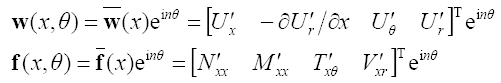where, in the above set of equations, N'x and M'xx are the force and moment resultants expressions as represented in the Figure 2. On the other hand, the variables of T' and V'xr are known as Kirchhaff parameters which are introduced in the Appendix E. In Eq. (7), prime symbol (') indicates that the term is divided by exp(iωt).

To consider the impact of structural damping in cylindrical shell, the Young's modulus is adopted as E = Ê(1 + i γ), where γ is the structural loss factor and Ê is a real coefficient of Young's modulus related to the material used in the cylinder.

## 3.2 Definition of Matrices ψ and φ

By defining certain interface metrics, it is possible to express the displacement and force vectors by the positive and negative going wave vectors, respectively. Hence, by substituting Eqs. (5) in Eq. (7) yields following relationship:

(8)where, ψ± and φ± are matrices of displacement vectors and force vectors, respectively. These relations can be written as follows:

(9)where, vectors of φj and ψj (j=1,...,8) as elements of ψ± and φ±, as follows:

(10)in which k = Eh(1-μ2)- 1 and D = Eh 3(12(1 - μ2)) are respectively membrane and bending stiffness of the shell. The relation between propagating wave vectors within the shell, e.g. a+ and b+ or b- and a- in the Figure 1, are made by the propagation matrices. The propagation matrix elements in the positive and negative direction, F+ , can be determined by the following relation:

(11)where, F+, introduced in Eq. (6), shows the propagation matrices in a cylinder with the length of x=L.

## 3.3 Definition of Reflection and Transmission Matrices

Taking into account the effects of flexible supports on the vibrations of cylindrical shell, it is necessary to define the stiffness matrix. This matrix in location is defined as follows:

(12)The diagonal elements (K)( x,r, θ,rθ) are the stiffness coefficients of springs which are extended evenly in arbitrary position on the cylinder. The diagonal shape of the matrix in Eq. (12) is appropriate for the linear vibration analysis of continuous systems, such as cylindrical shells.

### 3.3.1 Ended Supports

The boundary conditions at the right and left supports, depicted in the Figure 1, should be satisfied. In order to satisfy these boundary conditions one can write:

(13)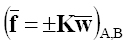According to Figure 1, the vectors of the incident wave amplitudes in the left and right boundaries are a- and b+, respectively. Moreover, the reflected waves vectors at these two boundaries are a+ and b-, respectively. Now the reflection matrix is constructed by Eqs. (8) and (13). In this regard, these equations were set based on the incident (a+, b+) and reflected waves (a-,b-) vectors as follows:

(14)where, RA,B are reflection matrices at the left and right borders of cylindrical shell, which can be defined by the following equations:

(15)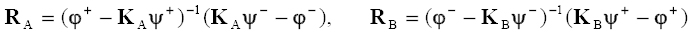It is clear that the set of equations of RA and RB in Eqs. (15) can satisfy the flexible boundary conditions for the both ends of the cylinder.

### 3.3.2 Wave Motion in Vicinity of Intermediate Supports and Shell Steps

In the analytical and semi-analytical solutions provided for the free vibrations of cylindrical shells, rarely can we find references on the flexible intermediate supports in the literature. The present sub section is intended to consider the effect of interior supports and shell steps on the vibration analysis of the cylindrical shell. Hence, Figure 3 shows incidence of a wave with a barrier including of a flexible support and a shell step.

Figure 3:
Waves motion in the vicinity of intermediate wave barriers of kind of shell step and intermediate support at same position

As seen in Figure 3, a part of the incident wave vector a+ i after colliding with the barrier is transferred b+ i , and some of it will be reflected a- i . Thus, considering the continuity conditions and the balance of forces in the barrier between the i th and the (i+1)th segments of the shell, we have:

(16)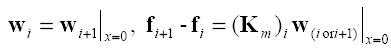in which (Km )i is the rigidity matrix of the i th intermediate support of the i th barrier that is a diagonal matrix including of elements of spring stiffness coefficients in the relevant independent directions, i.e. x, r ϑ, ϑ and r in Eq. (12). Using Eq. (8) in Eq. (16), and according to the equality of geometric and mechanical properties of the cylindrical shell in each segment, the following equality is concluded:

(17)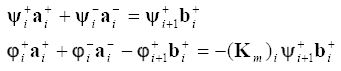Reflection and transmission matrices that connect the incident wave vector to the barrier, a+ i , transmitted vector, b+ i , and reflected vector, a- i , are defined as follows:

(18)where, ti,i+ 1 is wave transmission matrix from left to right, i.e., from the i th to the (i+1)th segments, and ri +1, i is the reflection matrix of the incident wave between the two mentioned segments. Using the results of Eq. (18) and rearranging Eq. (17), the reflection and transmission matrices can be obtained as:

(19)where, I is a four by four identity matrix. In a same manner, the reflection and transmission matrices in the opposite direction, i.e. right to left direction, can also be obtained.

# 4 EXTENDED VECTORIAL WAVE METHOD

In Figure 4, wave motion in a cylindrical shell with several elastic supports is shown. Accordingly, the relationship between the vectors of the output waves from the first intermediate support, i.e. b-1 and a+1, in terms of input waves vector to the support i.e. b+1 and, a-1 is as follows (it should be noted that for homogeneous materials like the present issue we have F+ = F- = F):

(20)Figure 4:
Waves motion in the vicinity of intermediate barriers of kind of intermediate supports and shell steps.

Moreover, by considering a+ 0 = RAa- 0 and a- 0 = F(L 1)b- 1 and using Eq. (20), we can write:

(21)where, Г+ 1, as a positive going inductor matrix, is defined as follows:

(22)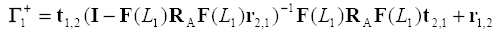In a same manner, the following relation can be given between a+ i and a- i for the i th intermediate support, i=2...n-1, as:

(23)where, the same as those given in Eq. (24), Г+ 2 to Г+ n -1 are defined as:

(24)Finally, in the n th segment of the shell, the following relations are established:

(25)The above equations consist of four equations with for unknown vectors, namely a+ n -1, a- n -1, b+ n , and b- n . Obviously, we can solve the sets of Eqs. (25) by each of these unknowns. For example, Eq. (25) can be solved by b+ n as follows:

(26)To achieve a non-trivial solution for free vibration analysis of the issue, using Eq. (26), the determinant of the coefficient of b+ n should be equal by zero. It should be noted that Eq. (26) is not a unique way for calculating the natural frequencies of the shell and we are able to write the same relations by the other wave vectors depicted in Figure 4. For example, in a backward approach, for the waves that are moving in the vicinity of the (n-1)th intermediated support (or the last intermediate support) of the shell, we have:

(27)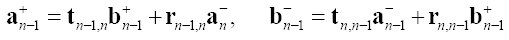Furthermore, in the n th segment or the last segment of the shell, we can write:

(28)considering b- n = RBb+ n and using Eq. (27) and (28), it gives a relation between b+ n- 1 and b- n- 1 as follows:

(29)where, Г- n -1 is a negative going inductor matrix, which is defined as:

(30)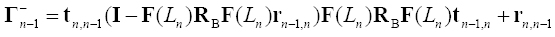It is possible to obtain a general relationship between b+ i and b- i for the i th intermediate support, i= 1, ..., n-2 as follows:

(31)where, Г- 1 to Г- n -2, are defined as follows:

(32)Wave motion in the first shell segment where depicted in the Figure 4 is considered here. By considering the canonical relationships between these wave vectors the following relations are governed:

(33)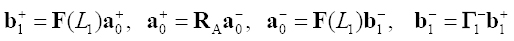The above set of equations consist of four equations with four unknowns, i.e. a+ 0, a- 0, b+ 1 and b- 1. For example, solving the above set of equations by a- 0 gives the following relation:

(34)For non-trivial results in the above equation, the determinant of the coefficient matrix of a- 0 must be zero, so we have:

(35)Invoking Eq. (35), all of the natural frequencies can be determined for a stepped shell with several intermediate supports with individual arbitrary properties for the applied supports. It is interesting to notice that both determinations represented in Eq. (26) or (35) provide a unique result for the frequency analysis of the shell. To achieve the roots of Eq. (35), Wittrick-Williams stemming algorithm can be used (Lee et al. (2007Lee, S.-K., Mase, B.R., Brennan, M.J. (2007). Wave propagation, reflection and transmission in curved Beams, Journal of Sound and Vibration 309:639-656.)). In addition, by normalizing the wave vector a- 0 and setting it from Eq. (34) in terms of the natural frequencies of the system, the mode shapes can be obtained.

# 5 FORCED VIBRATION

Concentrated actuating (or sensing) in the form of ring loading or point loading are applicable for several industrial tests, e.g. non-distractive tests and vibration control (Mei (2009Mei, C. (2009). Hybrid wave/mode active control of bending vibrations in beams based on the advanced Timoshenko theory, Journal of Sound and Vibration 322:29-38.), Hu et al. (2015Hu, S.D., Li, H., Tzou, H.S. (2015). Precision microscopic actuations of parabolic cylindrical shell reflectors, Journal of Vibration Acoust 137(1):011013, 1-11.), Jiang et al. (2012Jiang, J., Yue, H.H., Deng, Z.Q., Tzou, H.S. (2012) Cylindrical shell control with center- and corner-placed photostrictive skew-quad actuator systems. Journal of Vibration and Acoustics 134:024503, 1-5.), Kim et al. (2013Kim, H.S., Sohn, J.W., Jeon, J., Choi, S.-B. (2013). Reduction of the radiating sound of a submerged finite cylindrical shell structure by active vibration control, Sensors 13:2131-2147.) and Popov (2005Popov, A.V. (2005). An acoustic-emission technique of forced vibrations used for flaw detection in thin-walled pressure vessels. Russian Journal of Nondestructive Testing 41:154-157. Translated from Defektoskopiya, 41(3):27-31.)). Ring excitation as a line loading (Achenbach (2003Achenbach, J.D. (2003). Reciprocity in elastiodynamics, Cambridge University.)) is a harmonic loading in arbitrary position along with the axial direction of the shell and distributed on a circle around the shell. Indeed, the mode shapes of the shell can be excited distinctly using the response of the shell to the ring excitation. In the other hand, point load excitation enables us to excite all possible modes of the considered issue. In Figure 5, a stepped circular cylindrical shell is depicted with two ended- and several intermediate-supports and under a ring load excitation at middle of an arbitrary segment of the shell.

As a general case, it is possible to choose any arbitrary function, including concentration point loading, in the circumferential direction using Fourier expansions. Effects of the ring harmonic loading on structural responses of the shell, can be obtained using the wave motion analysis within the shell in the vicinity of the loading position. Continuity and force balancing between i th and (i+1)th segments of the shell exactly at the loading position are satisfied by the following relations:

(36)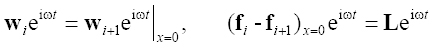where, indices i and i+1 in the above equation are referred to the left and right sides of the loading positions, respectively. Furthermore, L is the ring loading vector consists of force and moment loading in the relevant directions as follows:

(37)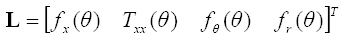where, fx,θ,r and Txx , are distributed forces and torque in the related directions, respectively. Substituting Eq. (8) in Eq. (36), it gives:

(38)or in the vector-matrix form it can be written as:

(39)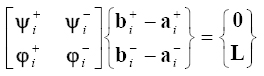where, a± i and b± i are the opposite going wave vector amplitudes at both sides of loading position, Figure 5. Solving the above equation yields:

(40)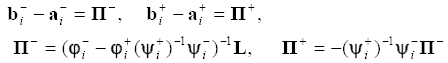From the wave motion analysis in the both sides of the loading position we can find:

(41)where, matrices of ℜi and ℜi+ 1 are defined as:

(42)By eliminating b+ i and a- i from Eqs. (40) and (41) we can write:

(43)Accordingly, for a certain load vector with arbitrary functions of ϑ, a+ i and b- i can be calculated from Eq. (43). Using Fourier expansion, arbitrary functions of ϑ can be provided for load vector, L. Accordingly we can use of the following relation:

(44)where, Ln is vector of Fourier parameters, and it can be obtained as follows:

(45)For modeling the point load excitation in arbitrary position on the shell, e.g. x=0 and θ = θ0 , in Figure 5, the point load can be expressed in terms of Dirac delta function:

(46)where,and, are amplitudes of distributed forces and torque in the relevant directions, respectively. Hence the Fourier coefficient parameters in the presence of point loading, Ln, can be obtained as follows:

(47)In the above equation, f 0 x,θ,r and T 0 xx , are the point forces and concentrated torques at θ0 , respectively. Hence, for point loading, superposing of circumferential mode numbers from zero to infinity is required. However, it is practically impossible to adopt all of the mode numbers, and hence, only finite numbers of n, or truncated n can be used for superposition depending on the required engineering accuracy. Using Eqs. (7), (8) and (10) and wave amplitudes obtained from Eq. (43), i.e. a+ i and b- i , for arbitrary distance, x, in the left or right sides of loading position, the amplitudes of the displacement fields in x, θ or r directions are obtained from the following relations:

(48)Accordingly, using Eqs. (43) and (48), all of displacement responses for the i th and (i+1)th segments of the shell can be obtained for arbitrary positions on i th and (i+1)th segments of the shell. In a same manner and by using Eqs. (23) and (31), similar relationships for the other segments of the shell can be founded easily.

# 6 NUMERICAL RESULTS

In this section, free and forced vibrations of stepped circular cylindrical shells affected by their supports characteristics, will be examined using the defined vector-matrix relations of VWM in the previous sections. In some possible cases, the results of VWM will be compared with the numerical results of FEM and the results available in the literature. Here, the following common geometrical and mechanical properties are adopted for the cases studies: the shell mean radius a=1 m, density ϱ=7800 kg/m3, Young modulus E=210 GPa and Poisson's ratio μ=0.3. Moreover, all results and charts are determined with the help of a self-written program in MATLAB. All of the obtained results are calculated with a PC with a dual core CPU of type of Intel Pentium G3220 (3.00GHz) and 3.39GB useable ram. However, as the code was not optimized for parallel computing, practically only one CPU of the computer was utilized in calculations.

To examine the effects of the support characteristics on natural frequencies and structural responses of the issue, certain types of flexible supports are utilized. The flexible supports are EI to EV, which are defined as follows:

EI , EII, EIII: Indicates that the support is rigid in the all directions except one flexible direction, i.e. r or ϑ or direction, respectively.

EIV , EV: Indicates that the support is free in the all directions except one flexible direction, i.e. r or ϑ direction, respectively.

Assuming very large stiffness for the springs at the supports (e.g. 1030) of the shell with flexible supports, the VWM with flexible supports reduces to VWM for a shell with rigid classical boundary conditions. For example, for the boundary conditions of Shear Diaphragm (SD) all the radial and circumferential stiffness should be considered as Kr= Kϑ ≈∞ and the rotational and axial stiffness should be equal to zero, i.e. Kx=0 and Krϑ =0, and so on.

## 6.1 Free Vibration Problem

### 6.1.1 Frequency Analysis

Tables 1 and 2 are regulated to compare the results of frequency analysis of a stepped shell with elastic ended supports using the proposed method and those reported in Qu et al. (2013Qu, Y., Chen, Y., Long, X., Hua, H., Meng, G. (2013). Free and forced vibration analysis of uniform and stepped circular cylindrical shells using a domain decomposition method, Applied Acoustics 74: 425-439.) and Chen et al. (2015Chen, M., Xie, K., Xu, K., Yu, P., Yan, Y. (2015). Wave based method for free and forced vibration analysis of cylindrical shells with discontinuity in thickness, ASME Journal of Vibration and Acoustics 107: 051004, 1-14.). Qu et al. (2013Qu, Y., Chen, Y., Long, X., Hua, H., Meng, G. (2013). Free and forced vibration analysis of uniform and stepped circular cylindrical shells using a domain decomposition method, Applied Acoustics 74: 425-439.) have solved the issue of free and forced vibration analysis in a stepped shell with flexible ended supports using a numerical approach, modified variational principle and least-squares weighted residual method mixed by domain decomposition method (DDM). In a same manner, Chen et al. (2015Chen, M., Xie, K., Xu, K., Yu, P., Yan, Y. (2015). Wave based method for free and forced vibration analysis of cylindrical shells with discontinuity in thickness, ASME Journal of Vibration and Acoustics 107: 051004, 1-14.) have utilized Flügge thin shell theory and the wave based method or WBM, and they have analyzed the same issue, adopted by Qu et al. (2013Qu, Y., Chen, Y., Long, X., Hua, H., Meng, G. (2013). Free and forced vibration analysis of uniform and stepped circular cylindrical shells using a domain decomposition method, Applied Acoustics 74: 425-439.), under a point load excitation. Hence, by considering the presence of only one step at the shell geometry and neglecting the interior supports, i.e. (Km )i = 0 in Eq. (19), as those in Qu et al. (2013Qu, Y., Chen, Y., Long, X., Hua, H., Meng, G. (2013). Free and forced vibration analysis of uniform and stepped circular cylindrical shells using a domain decomposition method, Applied Acoustics 74: 425-439.) and Chen et al. (2015Chen, M., Xie, K., Xu, K., Yu, P., Yan, Y. (2015). Wave based method for free and forced vibration analysis of cylindrical shells with discontinuity in thickness, ASME Journal of Vibration and Acoustics 107: 051004, 1-14.), a comparison between the results of VWM and the results reported by Qu et al. (2013Qu, Y., Chen, Y., Long, X., Hua, H., Meng, G. (2013). Free and forced vibration analysis of uniform and stepped circular cylindrical shells using a domain decomposition method, Applied Acoustics 74: 425-439.) and Chen et al. (2015Chen, M., Xie, K., Xu, K., Yu, P., Yan, Y. (2015). Wave based method for free and forced vibration analysis of cylindrical shells with discontinuity in thickness, ASME Journal of Vibration and Acoustics 107: 051004, 1-14.) is performed. The results of this comparison are summarized in Table 1. The symbol of m in Table 1 denotes the axial mode number. As it can be seen in Table 1, there is a good agreement between the results of VWM and the results available in literature for the case of a stepped shell with flexible supports.

Table 1:
Natural frequency factor (Ω) and EI-EI, EII-EII or EIII-EIII ended supports for a shell with one step (L 1 /L=0.5 and h 1 /a=0.01, h 2 /h 1=2; elastic support stiffness: K r = 5 × 107 N m-1 and K θ = 1.5 × 108 N m-1 and m=1).

Table 2:
Comparison of frequency factors for a three-stepped cylindrical shell with SD-SD ended supports (L 1 /L= L 2/L= L 3 /L= L 4 /L=0.25 and h 1 /a=0.01, h 2 /h 1=2, h 3 /h 1=3, h 4/h 1=4).

Table 2 shows the frequency analysis in free vibrations of a three stepped cylindrical shell with different axial lengths and SD-SD ended supports obtained by using VWM and the results obtained based on DDM by Qu et al. (2013Qu, Y., Chen, Y., Long, X., Hua, H., Meng, G. (2013). Free and forced vibration analysis of uniform and stepped circular cylindrical shells using a domain decomposition method, Applied Acoustics 74: 425-439.) and WBM by Chen et al. (2015Chen, M., Xie, K., Xu, K., Yu, P., Yan, Y. (2015). Wave based method for free and forced vibration analysis of cylindrical shells with discontinuity in thickness, ASME Journal of Vibration and Acoustics 107: 051004, 1-14.), respectively. By neglecting all flexible intermediate supports, namely (Km )i = 0 in Eq. (19), the present work is comparable with those reported by Qu et al. (2013Qu, Y., Chen, Y., Long, X., Hua, H., Meng, G. (2013). Free and forced vibration analysis of uniform and stepped circular cylindrical shells using a domain decomposition method, Applied Acoustics 74: 425-439.) and Chen et al. (2013Chen, Y., Jin, G., Liu, Z. (2013). Free vibration analysis of circular cylindrical shell with nonuniform elastic boundary conditions, International Journal of Mechanical Sciences 74: 120-132.). Despite of differences in shell theory used by Qu et al. (2013Qu, Y., Chen, Y., Long, X., Hua, H., Meng, G. (2013). Free and forced vibration analysis of uniform and stepped circular cylindrical shells using a domain decomposition method, Applied Acoustics 74: 425-439.), Table 2 indicates a good proximity between all results obtained by the proposed method and those given in the literature.

To examine the accuracy of the VWM in the presence of intermediate supports, the results of present method are compared with the results of Xiang et al. (2002Xiang, Y., Ma, Y.F., Kitipornchai, S. (2002). Exact solutions for vibration of cylindrical shells with intermediate ring supports, International Journal of Mechanical Sciences 44: 1907-1924.). In the study of Xiang et al. (2002Xiang, Y., Ma, Y.F., Kitipornchai, S. (2002). Exact solutions for vibration of cylindrical shells with intermediate ring supports, International Journal of Mechanical Sciences 44: 1907-1924.) presented an exact solution, a state-space technique (SST), to solve the problem of vibrations of a uniform circular cylindrical shell based on the Goldenveizer-Novozhilov shell theory by using different classic ended supports e.g. clamped (CL), SD, and free (F) supports and different numbers of intermediate rigid supports. In order to a possible comparison with the study of Xiang et al. (2002Xiang, Y., Ma, Y.F., Kitipornchai, S. (2002). Exact solutions for vibration of cylindrical shells with intermediate ring supports, International Journal of Mechanical Sciences 44: 1907-1924.), the thickness of the shell in the present study should be considered as uniform. Hence, the interface matrices of ψ and φ are required to be equal for each segment, i.e. ψ± 1 = a ψ± 2 = ...= ψ± n and φ± 1 = φ± 2 =...= φ± n in Eq. (19). In fact, Eqs. (3) and (10) all are independent from the shell lengths in each segment, and the lengths of the shell segments only appear in the propagation matrix relations. Comparison of the frequency factor obtained for a uniform shell by VWM and SST for diverse circumferential and axial mode numbers, i.e. n=1, ..., 3 and m=1,...,4, is made in Tables 3 and 4 in the presence of two and three numbers of intermediate RS, respectively. Despite the differences in the applied theory in this study and those utilized in the reference Xiang et al. (2002Xiang, Y., Ma, Y.F., Kitipornchai, S. (2002). Exact solutions for vibration of cylindrical shells with intermediate ring supports, International Journal of Mechanical Sciences 44: 1907-1924.), a very good proximity of the given results with both methodologies can be seen, especially in the case of thinner shells.

Table 3:
Comparison of frequency factor, Ω, for a shell with two intermediate ring supports and different thicknesses and ended supports conditions.

Table 4:
Comparison of frequency factor, Ω, for a shell with three intermediate ring supports and different thicknesses and ended supports conditions

In this direction, fundamental frequency factors, Ωf are represented in Table 5, given by VWM and SST applied in Xiang et al. (2002Xiang, Y., Ma, Y.F., Kitipornchai, S. (2002). Exact solutions for vibration of cylindrical shells with intermediate ring supports, International Journal of Mechanical Sciences 44: 1907-1924.) for different uniform shell lengths and thicknesses and in the presence of different numbers of intermediate RS. Again, a good proximity can be seen in the obtained results by the two methods applied.

Table 5:
Comparison of fundamental frequency factor, Ω f , in the presence of various numbers of intermediate RS.

Figures 6 show the effect of the wave barriers location on the natural frequencies of circular cylindrical shells. Here, the examined barriers are of type of shell step and intermediate radial supports (RS). In order to make a fair comparison, all conditions are considered the same as those given in Chen et al. (2015Chen, M., Xie, K., Xu, K., Yu, P., Yan, Y. (2015). Wave based method for free and forced vibration analysis of cylindrical shells with discontinuity in thickness, ASME Journal of Vibration and Acoustics 107: 051004, 1-14.). Hence, the geometrical and mechanical considerations in the presence and the absence of the intermediate support are as follows: the thickness ratio h 1/a=0.01, the step thickness ratio h 1/h 2=0.25, 0.5, 2, and 4, the length to radius ratio L/a=5, and 10 and finally step location (and intermediate support location) is at L 1/L=0.5. Figures 6 show that the obtained results in the absence of the intermediate support have good agreement with those reported in the literature (Chen et al. (2015Chen, M., Xie, K., Xu, K., Yu, P., Yan, Y. (2015). Wave based method for free and forced vibration analysis of cylindrical shells with discontinuity in thickness, ASME Journal of Vibration and Acoustics 107: 051004, 1-14.)).

Figure 6:
Beam mode- (a,c) and fundamental-frequency parameters (b,d) versus the location of the wave barrier, L 1/L, for a SD-SD shell with (------) and without (____) intermediate support -□- h 1/h 2=2, -○- h 1/h 2=0.5, - Δ- h 1/h 2=4, and-◊- h 1/h 2=0.25 including geometrical characteristics of: (a,b) L/a=5 and (c,d) L/a=10.

Great impact of the existence of interior support on increasing of both beam mode- and fundamental-frequency factors is clearly visible in the all cases considered for Figures 6. It is a very logical phenomena where happened due to the increase of the whole system rigidity, affected by the applied interior support. Hence, depending on the thickness ratio h 2/h 1, the greatest variation of all frequencies approximately, occurs for the intermediate type of RS, applied in an interval of 0.4<L 1/L<0.6. As it can be seen in these figures, the maximum fundamental frequency factor for all of the shell length ratios, i.e. L/a=5 and 10, in the presence of intermediate RS, happened in thickness ratio of h 2/h 1=0.25 and in the position ratio of L 1/L=0.4. However, in the case of maximum beam mode frequency factor, depending on the shell length ratio, it happens in thickness ratio of h 2/h 1=0.25 for L/a=10 or thickness ratio of h 2/h 1=0.5 for L/a=5.

### 6.1.2 Mode Shapes

Using Eq. (26) or (34), it is possible to obtain all mode shapes of the cylindrical shell. In this section, radial mode shapes for beam modes, i.e. n=m=1, in the axial directions of a shell with different numbers of intermediate RS and SD-SD ended supports are plotted in Figures 7 to 9 (By these considerations we are able to tracing satisfaction of the support conditions and continuity, simultaneously). Two cases for examination their mode shapes are considered here. In the first case a uniform shell and in the next case a stepped shell with different numbers of intermediate ring supports are considered here.

Figure 7:
Mode shapes of a homogeneous SD-SD shell with three intermediate ring supports for m=n=1 (beam mode) and in different conditions as: h/a=0.01, 0.05, 0.1 a) L/a=10 b) L/a=5.

Figure 8:
Mode shapes corresponding with beam mode of the shell with h/a=0.05 and SD-SD ended supports in three cases of numbers of applied intermediate RS and two shell lengths of a) L/a=10 b) L/a=5.

Figure 9:
Mode shapes of a shell corresponding with beam mode (n=m=1) and shell lengths of a,c,e) L/a=10 and b,d,f) L/a=5: a,b) One (at L 1 /L=0.5) c,d) two (at L 1 /L= L 3 /L=0.25) and e,f) three (at L 1 /L= L 2 /L= L 3 /L=0.25) intermediate supports in three different cases of stepped shells.

In the case of a uniform shell, the effects of shell thickness on mode shapes corresponding with beam mode frequencies are depicted in Figures 7 for two shell lengths, i.e. L/a=10 and 5, respectively. In this figure, the normalized radial displacement are plotted for h/a=0.01, 0.05 and 0.1 in the presence of three numbers of intermediate RS. Due to the geometry and support condition chosen for the issue, symmetrical mode shapes are expected. As can be seen in these figures, the slope of the radial displacement fields, i.e. dUr /dx, at all support positions decreases in the thicker and shorter cylindrical shells. Also, the shorter cylinders have higher sensitivity to the shell thickness.

The mode shapes of the shell with different numbers of intermediate RS corresponding with beam mode frequencies are plotted in Figures 8 for two shell lengths, i.e. L/a=10 and 5, respectively. Hence, three cases are considered in these figures. Case 1-1: one intermediate RS at L 1/L =0.25, Case 2-1: two numbers of intermediate RS at L 1/L = L 2/L =0.25, and Case 3-1: three numbers of intermediate RS at L 1/L = L 2/L = L 3/L =0.25. In these figures, satisfying of continuity and all the support conditions applied to such unsymmetrical conditions can be seen obviously in the all given results.

In Figures 9a to 9f, radial mode shapes are depicted in the axial direction of a stepped shell with different combinations of intermediate ring supports. Hence, three cases of shell thickness and steppes are considered as: Case 1-2: uniform Shell, Case 2-2: three steppes in the shell; L 1/L= L 2/L =L 3/ L=0.25 and h 1/a=0.01, h 2/h 1=2, h 3/h 2=3 and h 4/h 3=4 and finally Case 3-2: with same condition by case 2-2 except thicknesses of h 2/h 1=3, h 3/h 2=5 and h 4/h 3=7. In Figures 9a, 9c and 9e total shell length is L/a=5 and shell length that considered in Figures 9b, 9d and 9f is L/a=5. Number of the interior supports used in these figures is respectively as one interior support at L 1/L=0.5 in Figures 9a and 9b, two interior ring supports at L 1/L=L 3/L=0.25 in Figures 9c and 9d and three interior supports at L 1/L= L 2/L= L 3/L=0.25 in Figures 9e and 9f. These figures indicate that continuity and support conditions of the issue are completely satisfied by the proposed method, simultaneously. However by changing the shell thickness from case 1-2 to case 3-2, radial deformations in normalized modes are graded and mostly tended to the thinner sides of the shell.

## 6.2 Forced Vibration

In this section a stepped circular cylindrical shell with SD-SD ended supports and three intermediate supports of different types is considered. Other considerations are: Length ratio, L/a=5, thickness ratios h 1/a=0.01 and h 2/h 1=2, h 3/h 2=2, h 4/h 3=2. In all of the following figures, the amplitude of the point load excitation is considered 100 N. The point loading are applied on the shell at θ0 = 0º and x/L=1/8 imposed at thinnest segments of the shell. In all graphs of frequency responses, frequency ranges are considered from 100 to 500 Hz and their steps are 2 Hz.

### 6.2.1 Verification of VWM Results

To achieve accurate results with low calculation time, minimum numbers of truncation of circumferential mode number, n, which was described in Sec. 6, is required to be determined. Figures 10 depicts the radial and circumferential displacement responses due to excitation of a point load (fr 0 100N) exactly at the middle of the first segments of the shell (x 1=L 1 /2 in Figure 5) in the radial direction. The results of these figures are plotted for some different truncation of n. In these figures, a good convergent results for all truncations, i.e. n=20, 30 and 40, is clearly visible. However, the differences between results of n=30 and 40 are negligible, and hence, n=30 is chosen for evaluating and plotting the Frequency Response Functions (FRF). Using VWM and with the non-optimized self-writing code, the mean elapsed time for calculation when n=30 was about 12.09 seconds.

Figure 10:
Convergency examination of structural radial and axial responses of a stepped shell with three intermediate ring supports at L 1/L= L 2/L= L 3/L=0.25 and shell thickness h 1/a=0.01 and h 2/h 1=2, h 3/h 2=2, h 4/h 3=2 and shell length of L/a=5 using VWM (a,b) and FEM (c,d) and comparing them (e,f).

Due to lack of proper comparison results in the literature to evaluate the required VWM solution time and its numerical performance, we have utilized the finite element method provided by ANSYS for validation and evalution of the results. The forced vibration analysis given by VWM for classical ended and intermediate supports, i.e. SD and RS is considered. Then, utilizing ANSYS, an excited shell with a point load at the middle of the first segment as the thinnest segment of the stepped shell is modeled using the four node elements of shell 63.

Among several compositions of mapped meshing, three different meshing styles are chosen for evaluation of FEM convergence. These cases are: Case 1-3: 60×100, Case 2-3:80×120 and Case 3-3: 100×140 nodes in the ϑ and x directions, respectively. The results of ANSYS for different grid sizes of cases 1-3 to 3-3 are plotted in Figures 10c and 10d. As it can be seen from Figures 10c and 10d, cases 2-3 and 3-3 are convergent and approximately can cover all possible peaks correspond with all the natural frequencies. In an overall comparison between the results of VWM and the results of ANSYS as a FEM, the results of both methods are in good agreement in the case 2-3 for both of the radial and axial displacement responses. These comparisons between VWM and FEM are made in the Figures 10e and 10f. The computational time for calculations of case 2-3 was about 32 minutes using two CPU cores where is approximately 159 times greater than those given by VWM, utilizing only one CPU core.

Stepped shell response to the radial and axial point load excitations at x 1/L 1=0.5 is evaluated in Figures 11 in the presence of three flexible intermediate supports of kind of EIV, illustrated in Figure 5. Hence, in the Figures 11, different stiffness values Kr =109, 1010 and 1030 N/m are considered for the applied intermediate supports and radial and axial responses to the excitations are plotted. One of these values, i.e. 1030 N/m, are corresponds to the radial rigid support (or RS), respectively, and hence, it is possible to trace the FRF changes in line with changes of the support characteristics. From these figures, depends of radial or axial excitations the following results can be concluded: as the stiffness of the intermediate supports decreased, axial amplitude, Ux , is more sensitive to the radial excitation and vice versa radial amplitudes, Ur , is more affected by the axial excitation. From these figures, reduction of both radial and axial amplitudes concluded. However, the obtained results for most of peaks show infinitesimal changes in the peak positions. Figures 11a-11d depict that some of the peaks in both radial and axial directions are vanished or reduced strongly in the case of Kr =107 N/m. It is a promising outcome as it indicates that the external excitation can be imposed safely in a certain limited range of frequency changes (without any resonance problem) by proper setting of the support stiffness. However, it should be noted that the problem of instability in the shell vibrations with the flexible supports could be an important issue that is not considered in the present study.

Figure 11:
Structural responses of a stepped shell with three flexible intermediate supports and SD-SD ended supports for a) radial and b) axial displacement amplitudes for radial excitation and c) radial and d) axial displacement amplitudes for axial excitation at the point load position.

In Figures 12, the effect of the position of EIV flexible supports on the structural response of the shell is depicted when Kr =108 N/m. Here, in order to examine the effect of the support positions, four different cases are considered. In the cases examined, all of the interior supports are RS, except: Case 1-4: without flexible support, Case 2-4: the left side interior support is flexible, Case 3-4: the middle interior support is flexible, and Case 4-4: the right side interior support is flexible.

Figure 12:
Structural responses of a stepped shell with three flexible intermediate supports and SD-SD ended supports for a) radial and b) axial displacement amplitudes for radial excitation and c) radial and d) axial displacement amplitudes for axial excitation at the point load positions.

Figures 12a and 12b illustrate radial and axial responses of the shell under radial excitation, respectively. In a same manner, the radial and axial responses of the shell under axial excitation are illustrated in Figures 12c and 12d, respectively. In an overall conclusion from these figures the following results can be concluded: the effect of the support characteristics on the structural radial and axial responses of the shell is directly related to the distance of the support position to the excitation position. It is interesting that the axial shell responses are more sensitive to the characteristics of remote supports in the presence of the radial excitations. Vice versa, in the presence of axial excitations, the radially shell response is more sensitive to the characteristics of the remote supports from the loading location, i.e. the supports that are positioned far from the loading location. This conclusion is important for non-destructive tests (NDT) purposes and fault detection of the applied supports.

### 6.2.3 Distributed Damping Effects

The effect of the structural damping, in the form of distributed damping type, on the radial and axial displacement response is depicted in Figures 13a and 13b. From these figures, it is clear that the amplitudes of peaks decreases proportional to the magnitude of the structural loss factor γ. However, the corresponding frequencies of the peaks do not show any significant dependency on γ and almost the peaks are independent of γ. However, in elsewhere except peak positions, the shell amplitudes, approximately, are independent of the structural loss factors, and they are not under significant influence of γ in forced vibration of the shell.

Figure 13:
Structural responses of a stepped shell with three flexible intermediate supports in the (a) radial and (b) axial directions of the shell and for different structural damping considerations.

# 7 CONCLUSION

The main objective of this paper was proposing an extensible method for analysis of free and force vibration of shells with arbitrary numbers of steps and flexible intermediate supports. To model the issue and based on Flügge thin shell theory, a stepped circular cylindrical shell was considered with two ended- and many intermediate-supports, which all of them are flexible with independent characteristics. The support conditions as well as the continuity of the stepped shell were satisfied accurately by introducing wave vectors going in the positive-negative direction along with the shell axis and determining relevant reflection and propagation matrices.

Based on VWM, contiguous and extensible matrix-kind relations were proposed for free and forced vibration analysis of the issue where contained of 4×1 vectors and 4×4 matrices independent of the number of steps and intermediate supports utilized. For free vibration analysis of the shell, the proposed method was successfully employed to obtain the respective natural frequencies of the system. Furthermore, the shell structural responses were investigated under point load excitations. The obtained results were successfully verified against those available in the literature and those obtained by FEM. The results of the present study in free and forced vibrations of the shell can be summarized as follows:

1. Structural responses of a vibrating shell are highly affected by the properties of its intermediate supports, and ignoring this important finding by using simplifying assumptions (classical supports) can lead to significant errors in prediction of vibrational behavior of the issue.

2. According to the obtained results, the proposed method, as an analytical method, provides accurate results in both free and forced vibrations of the shell without any limitation in properties and numbers of the steps or the flexible intermediate supports which were applied.

3. In an overall comparison, the proposed method shows a great numerical performance versus FEM especially in the case of point load excitations of the issue. In this case, although a great numbers of truncated modes is needed to be superposed, the required computational time by VWM was about 159 times less than that given by FEM ANSYS.

4. The obtained results show that the axial (radial) shell responses are more sensitive to the characteristics of remote supports in the presence of radial (axial) excitations. This could be an important conclusion for fault detection of the applied supports.

It should be noted at this end that the proposed method is fully capable for applying to accurate design of several flexible supports with arbitrary properties for a certain stepped cylindrical shell along with the shell axis. We believe that the proposed method can be extended and tackles complicated issues such as non-homogeneous shells and shells made of composite layers or functionally graded materials. The present method also seems capable of being applied as a powerful basis for control of the shell vibration problems and predicting and verifying the NDT results which can be subject of future studies.

# References

• Achenbach, J.D. (2003). Reciprocity in elastiodynamics, Cambridge University.
• Bahrami, A., Ilkhani, M.R., Nikkhah-Bahram, M. (2012). Wave propagation technique for free vibration analysis of annular circular and sectorial membranes. Journal of Vibration and Control 0:1-7.
• Chen, M., Xie, K., Xu, K., Yu, P., Yan, Y. (2015). Wave based method for free and forced vibration analysis of cylindrical shells with discontinuity in thickness, ASME Journal of Vibration and Acoustics 107: 051004, 1-14.
• Chen, Y., Jin, G., Liu, Z. (2013). Free vibration analysis of circular cylindrical shell with nonuniform elastic boundary conditions, International Journal of Mechanical Sciences 74: 120-132.
• Hagedorn, P., Das-Gupta, A. (2007). Vibrations and waves in continuous mechanical systems, John Wiley & Sons Ltd, England.
• Hu, S.D., Li, H., Tzou, H.S. (2015). Precision microscopic actuations of parabolic cylindrical shell reflectors, Journal of Vibration Acoust 137(1):011013, 1-11.
• Huang, D., Tang, L., Cao, R. (2013). Free vibration analysis of planar rotating rings by wave propagation. Journal of Sound and Vibration 332:4979-4997.
• Jiang, J., Yue, H.H., Deng, Z.Q., Tzou, H.S. (2012) Cylindrical shell control with center- and corner-placed photostrictive skew-quad actuator systems. Journal of Vibration and Acoustics 134:024503, 1-5.
• Karczub, D.G. (2006). Expression for direct evaluation of wave number in cylindrical shell vibration studies using the Flügge equation of motion, Journal of the Acoustical Society of America 119:3553-3557.
• Kim, H.S., Sohn, J.W., Jeon, J., Choi, S.-B. (2013). Reduction of the radiating sound of a submerged finite cylindrical shell structure by active vibration control, Sensors 13:2131-2147.
• Lee, S.-K., Mase, B.R., Brennan, M.J. (2007). Wave propagation, reflection and transmission in curved Beams, Journal of Sound and Vibration 309:639-656.
• Loy, C.T., Lam, K.Y. (1997). Vibration of cylindrical shells with ring support, International Journal of Impact Engineering, 35 (1997) 455-471.
• Ma, Y., Zhang, Y., Kennedy, D. (2015). A symplectic analytical wave based method for the wave propagation and steady state forced vibration of rectangular thin plates, Journal of Sound and Vibration 339:196-214.
• Mace, B.R. (1984). Wave reflection and transmission in beams, Journal of sound and vibration, 97(2):237-246.
• Mei, C. (2009). Hybrid wave/mode active control of bending vibrations in beams based on the advanced Timoshenko theory, Journal of Sound and Vibration 322:29-38.
• Mei, C. (2016). Experimental validation of wave vibration analysis of complex vibrations in a two-story metallic space frame based on the Timoshenko bending theory. ASME Journal of Vibration and Acoustics 138(2):021003.
• Mei, C., Mase, B.R. (2005). Wave reflection and transmission in Timoshenko beams and wave analysis of Timoshenko beam structures. Journal of Vibration and Acoustics 127:382-394.
• Nikkhah-Bahrami, M., Khoshbayani-Arani, M., Rasekh-Saleh, N. (2011). Modified wave approach for calculation of natural frequencies and mode shapes in arbitrary non-uniform beams, Scientia Iranica 18(B):1088-1094.
• Popov, A.V. (2005). An acoustic-emission technique of forced vibrations used for flaw detection in thin-walled pressure vessels. Russian Journal of Nondestructive Testing 41:154-157. Translated from Defektoskopiya, 41(3):27-31.
• Qatu, M.S. (1989-2000). Recent research advances In the dynamic behavior of shells, (2002) Part 2: Homogeneous Shells. ASME Applied Mechanics Reviews, 55: 415-434.
• Qu, Y., Chen, Y., Long, X., Hua, H., Meng, G. (2013). Free and forced vibration analysis of uniform and stepped circular cylindrical shells using a domain decomposition method, Applied Acoustics 74: 425-439.
• Renno, J.M., Mace, B.R. (2011). Calculating the forced response of two-dimensional homogeneous media using the wave and finite element method, Journal of sound and vibration, 330: 5913-5927.
• Salahifar, R., Mohareb, M. (2012). Finite element for cylindrical thin shells under harmonic forces, Finite Elements in Analysis and Design, 52: 83-92.
• T. Loy, C., Y. Lam, K., Shu, C. (1997). Analysis of cylindrical shells using generalized differential quadrature, Shock and Vibration 4: 193-198.
• Wang, C. M., Swaddiwuhipong, S., Tian, J. (1997). Ritz method for vibration analysis of cylindrical shells with ring stiffeners, Journal of Engineering Mechanics, 123(2):134-142.
• Xiang, Y., Ma, Y.F., Kitipornchai, S. (2002). Exact solutions for vibration of cylindrical shells with intermediate ring supports, International Journal of Mechanical Sciences 44: 1907-1924.
• Zhang, L., Xiang, Y. (2006). Vibration of open circular cylindrical shells with intermediate ring supports, International Journal of Solids and Structures 43: 3705-3722.
• Zhang, L., Xiang, Y. (2007). Exact solution for vibration of stepped circular cylindrical shells, Journal of sound and vibration 299: 948-964.
• Zhou, H., Li, W., Lv, B., Li, W.L. (2012). Free vibration of cylindrical shells with elastic-support boundary conditions, Applied Acoustics 73: 751-756.
• Zhou, J., Yang, B. (1995). Distributed transfer function method for analysis of cylindrical shells, AIAA Journal 33: 1698-1708.

# APPENDIX A

Differential operators of the equation of motion:

(A.1)where in the above equations, cL =(E/ϱ(1-μ 2))0.5 is the speed of dispersion of longitudinal waves and η=h/a(12)0.5 is a dimensionless coefficient.

# APPENDIX B. COEFFICIENTS OF ELEMENTS OF THE MATRIX [χ]

The coefficients of Eq. (3) are as follows:

(B.1)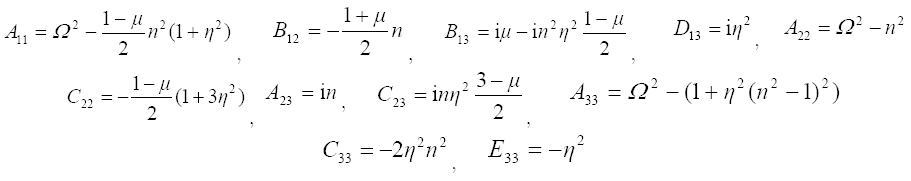# APPENDIX C. COEFFICIENTS OF THE POLYNOMIAL EQUATION

Coefficients Ai (i=0,2,4,6,8) presented in Eq. (4) are given as bellow

(C.1)# APPENDIX D. THE WAVE NUMBER AND FREQUENCY ANALYSIS

The roots of the Eq. (4) represent the behavior of the elastodynamic wave propagation in the shell to the frequency changes. In this regard, four out of eight possible roots of wave numbers obtained are related to positive going waves and in contrast four other remaining roots are associated with negative going waves. From these roots, four different types of waves can be detected, depending on the combination of the real and imaginary values of roots as (Zhou wt al. (2012Zhou, H., Li, W., Lv, B., Li, W.L. (2012). Free vibration of cylindrical shells with elastic-support boundary conditions, Applied Acoustics 73: 751-756.) and Karczub (2006Karczub, D.G. (2006). Expression for direct evaluation of wave number in cylindrical shell vibration studies using the Flügge equation of motion, Journal of the Acoustical Society of America 119:3553-3557.)):

Type 1: Λ = ±ζ1 (Propagating waves), Type 2: Λ = ±ζ2 (evanescent waves)

Type 3: Λ = ±(ζ3 +4) (Standing waves), Type 4: Λ = ± (ζ3 -4) (Standing waves)

Where ζi (i = 1, ..., 4) are real values. All eight dimensionless wave number are defined as the roots of the Eq. (4) independently as follows:

(D.1)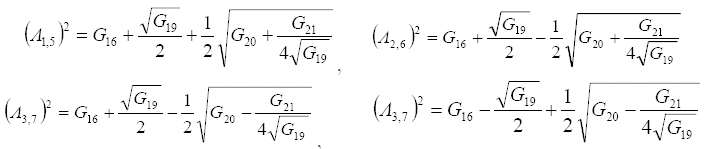where the coefficients Gi (i=1,... ,21) are provided in the follows. Generally, in the absence of damping in vibration of the cylindrical shells, all the four types of waves, i.e., propagating, decaying and standing type 3 and 4, can be created in different frequency ranges. The parameters used in the Eqs. (D.1) are defined as:

(D.2)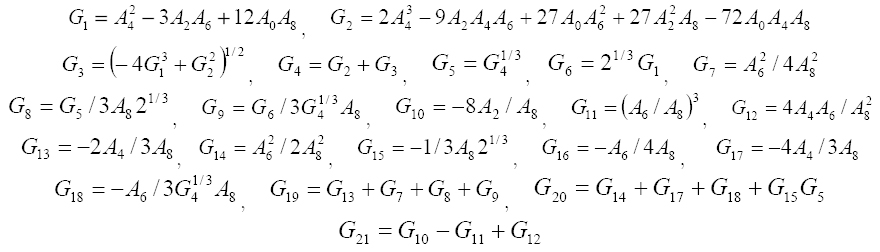# APPENDIX E

Force and momentum resultants:

(E.1)# Publication Dates

• Publication in this collection
Nov 2016